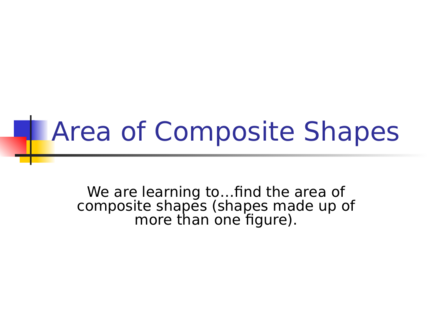# Area of Composite FiguresContributed by:We are learning to find the area of composite shapes (shapes made up of more than one figure).
1. Area of Composite Shapes
We are learning to…find the area of
composite shapes (shapes made up of
more than one figure).
2. Area of Composite Shapes
Example #1: Find the area of the composite shape
below.
Plan:
Area of a + Area of ½ Circle
Rectangle l(w)
4 feet + ½ πr2
4(10)+ ½ π(2)2
4(10) + ½ π(4)
10 feet
Diameter = 4 40 + ½ π(4)
feet
Radius = 2 feet 40 + (1.57)(4)
40 + 6.28
46.28 square
feet
3. Area of Composite Shapes
Example #2: Find the area of the shaded
Plan:
Area of a Square- Area of Circle
l(w)- πr2
4 cm
4 cm 4(4) - π(2)2
4 cm
4(4) - π(4)
16 - π(4)
4 cm 16 - 12.56
3.44 square cm
4. Area of Composite Shapes
Example #3: Find the area of the shaded
Plan:
6 in Area of a - Area of a Triangle
Trapezoid
½(h)(b1 + b2) - ½(b)(h)
4 in ½(4)(6 + 12)- ½(6)(4)
5 in ½(4)(18) - ½(6)(4)
36 - ½(6)(4)
36 - 12
24 square inches
12 in
5. Area of Composite Shapes
Example #4: Find the area of the shaded
region.
Plan:
15 ft
Area of a - Area of a Circle
Trapezoid 2
½(h)(b1 + b2)- πr
8 ft ½(8)(6 + 15)- π(4)2
10 ft
½(8)(21) - π(4)2
½(8)(21) - π(16)
6 ft 84 - π(16)
84 – 50.24
33.76 square feet
6. Area of Composite Shapes
Example #5: Find the area of the polygon
below.
9 inches Plan:
Area of a + Area of a
Rectanglel(w)+ l(w)Rectangle
9(18 + 5(11)
in )
162 + 55
11 inches 217 Square inches
5
in